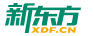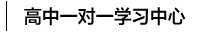#400-817-1616

## 导数公式

来源：网络

时间： 2018-11-16 17:01

1.y=c(c为常数)y‘=0

2.y=x^ny’=nx^(n-1)

3.y=a^xy‘=a^xlna

y=e^xy’=e^x

4.y=logaxy‘=logae/x

y=lnxy’=1/x

5.y=sinxy‘=cosx

6.y=cosxy’=-sinx

7.y=tanxy‘=1/cos^2x

8.y=cotxy’=-1/sin^2x

9.y=arcsinxy‘=1/√1-x^2

10.y=arccosxy’=-1/√1-x^2

11.y=arctanxy‘=1/1+x^2

12.y=arccotxy’=-1/1+x^2

（责任编辑：刘汉甜）